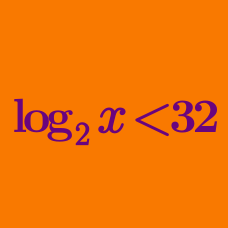Algebra

# Logarithmic Inequalities - Same Base

Solve the logarithmic inequality $\log_3(x-9)+\log_3(x-7)<1.$

How many integer solutions does the following inequality have: $\log_{3}x^{9}+\left( \log_{3}x \right)^2 \le 10?$

If the solution to the inequality $(\log_{5} x)^2 < 5 - \log_{5} x^{4}$ is $a < x < b$, what is the value of $\frac{1}{ab}$?

Solve the logarithmic inequality $\log_2 (15x-17) \ge \log_2 (x+16).$

How many positive integers $x$ satisfy the inequality $\log_{0.1} (x-15) - \log_{0.1} (301-x) > 0$?

×